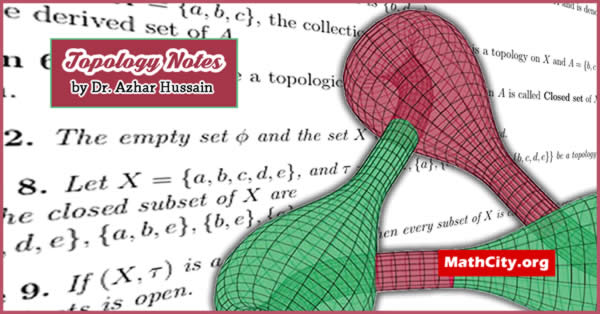# General Topology by Azhar HussainThe area of topology known as general topology (also known as point set topology) is concerned with the fundamental concepts and constructs of set theory utilised in topology. Most other fields of topology, such as differential topology, geometric topology, and algebraic topology, are built upon it. The three key ideas of point-set topology are connectedness, compactness, and continuity. Continuous functions move points from one location to another. Compact sets are those that can be completely covered by a small number of sets of any size. Connected sets are sets that cannot be split into two far-reaching portions. Using the idea of open sets, the concepts “nearby,” “arbitrarily small,” and “far apart” can all be clarified.

 Name Lecture Notes on General Topology Dr. Azhar Hussain 20 pages PDF 254 KB
• Definition
• Examples
• Neighborhood of point
• Accumulation point
• Derived Set
• Interion of set
• Exterior of set
• Neighborhood System of a point
• Base for a Topology
• and related theorem

• notes/general-topology-azhar-hussain